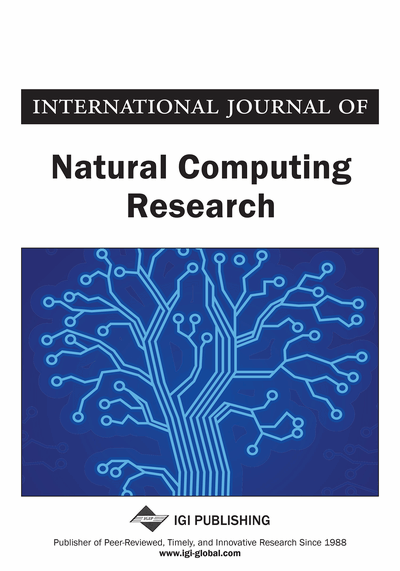# A Complexity-Invariant Measure Based on Fractal Dimension for Time Series Classification

Ronaldo C. Prati (Centro de Matemática, Computação e Cognição, Universidade Federal do ABC, Santo André, SP, Brazil) and Gustavo E. A. P. A. Batista (Instituto de Ciências Matemáticas e de Computação, Universidade de São Paulo, São Carlos, SP, Brazil)
DOI: 10.4018/jncr.2012070104
Available
\$37.50
No Current Special Offers

## Abstract

Classification is an important task in time series mining. It is often reported in the literature that nearest neighbor classifiers perform quite well in time series classification, especially if the distance measure properly deals with invariances required by the domain. Complexity invariance was recently introduced, aiming to compensate from a bias towards classes with simple time series representatives in nearest neighbor classification. To this end, a complexity correcting factor based on the ratio of the more complex to the simpler series was proposed. The original formulation uses the length of the rectified time series to estimate its complexity. In this paper the authors investigate an alternative complexity estimate, based on fractal dimension. Results show that this alternative is very competitive with the original proposal, and has a broader application as it does neither depend on the number of points in the series nor on a previous normalization. Furthermore, these results also verify, using a different formulation, the validity of complexity invariance in time series classification.
Article Preview
Top

## 1. Introduction

Time series data are being generated by numerous applications, with domains ranging from medical and biological experimental observations (e.g. electro encephalograms and cardiograms), financial and trade transactions recordings (e.g. stock market daily fluctuations, periodic variations of mercantile prices of goods and services), data from dynamic processes in scientific experiments (e.g. data generated by the Large Hadron Collider and signals recorded by radio telescopes) and readings of sensor networks (e.g. recordings from high precision agriculture sensors and advanced video game movement control tracking), to name but a few. This ubiquity has propelled the interest in time series data mining in recent years.

In the case of time series classification, numerous research papers report empirical evidence that a simple nearest neighbor classification approach is very competitive, and often superior to many state of the art classification methods (Ding et al., 2008). This is especially true if the distance measure properly captures the “invariances” required by the domain at hand (Batista et al., 2011). For instance, some domains require invariance to “warping” (local non-linear accelerations and decelerations). This is the case of most motion capture applications, where the technique used for measuring similarity can compensate from (sub) sequences which may vary in time or speed; similarities in movement patterns would be detected even if in one video the subject was moving slowly and in another it is moving quicker, or even if there were accelerations and decelerations during the course of one observation. The Dynamic Time Warping () technique (Berndt & Clifford, 1994) is a well known and widely used method which allows the time series to be “stretched'' or “compressed'' to provide a better match with another time series. Other invariances include uniform scaling, offset, amplitude scaling, phase, occlusion, uncertainty and wandering baseline. Several methods have been proposed to handle them - see (Batista et al., 2011) for a thoughtful discussion about invariances and methods to handle invariances in time series distance calculation.

Batista et al. (2011) introduced new kind of invariance. This invariance was called complexity invariance by the authors. The idea is rather simple and appealing: the “complexity'' of a time series may introduce a bias in nearest neighbor classification because complex time series tend to be “closer” to simple time series rather than to other complex time series if the distance measure is not compensated for complexity. Therefore, if a class has simpler time series representations than others, this class will be preferred in nearest neighbor classification and introduce a bias towards the class with simpler representatives. The authors intuitively defined the complexity of a series as “having many peaks, valleys and features”. To capture the notion of complexity difference between two time series, they propose a complexity correction factor based on the ratio of the lengths between the longest (the more complex) to the shortest (simpler) “rectified'' series. This correction factor is then multiplied by the distance between the two series in order to make it “complexity-invariant'.

Experimental evidence reported in their paper shows the suitability of the proposed complex invariance correction in numerous domains. However, as the authors report in their paper, the correcting factor used in the experiments is an ad-hoc formulation, and is one of possible complexity measures empirically chosen.

In this paper, we evaluate the use of an alternative way to estimate complexity of time series based on fractal dimension. The fractal (or Hausdorff) dimension is a measure of roughness (or smoothness) for time series and spatial data. As the fractal dimension is an intrinsic measure of the series, it does not depend on the number of the observations of the time series, nor a previous normalization step of amplitude and offset, as the original formulation does.

## Complete Article List

Search this Journal:
Reset
Open Access Articles: Forthcoming
Volume 11: 4 Issues (2022): Forthcoming, Available for Pre-Order
Volume 10: 4 Issues (2021): 3 Released, 1 Forthcoming
Volume 9: 4 Issues (2020)
Volume 8: 4 Issues (2019)
Volume 7: 4 Issues (2018)
Volume 6: 2 Issues (2017)
Volume 5: 4 Issues (2015)
Volume 4: 4 Issues (2014)
Volume 3: 4 Issues (2012)
Volume 2: 4 Issues (2011)
Volume 1: 4 Issues (2010)
View Complete Journal Contents Listing